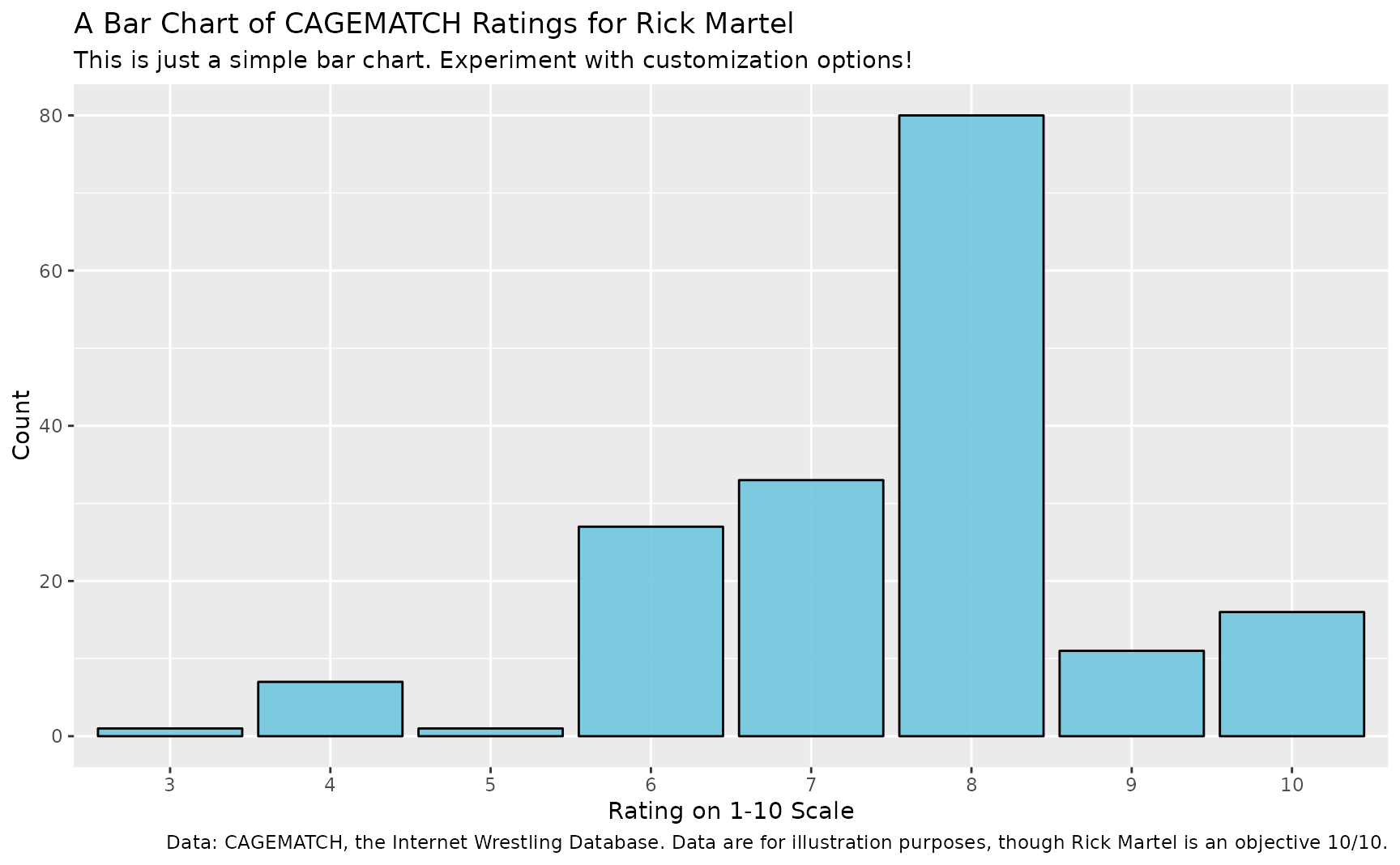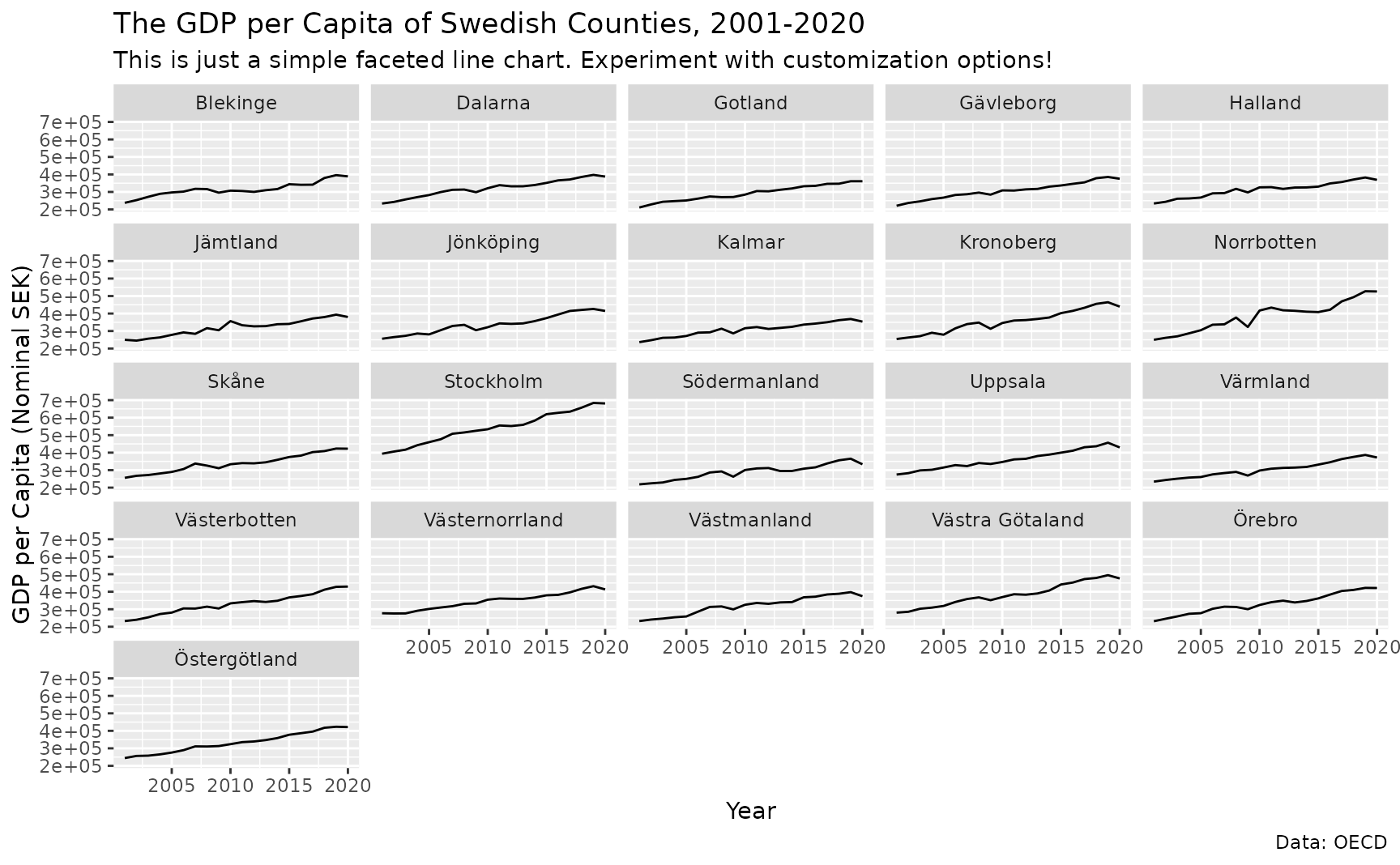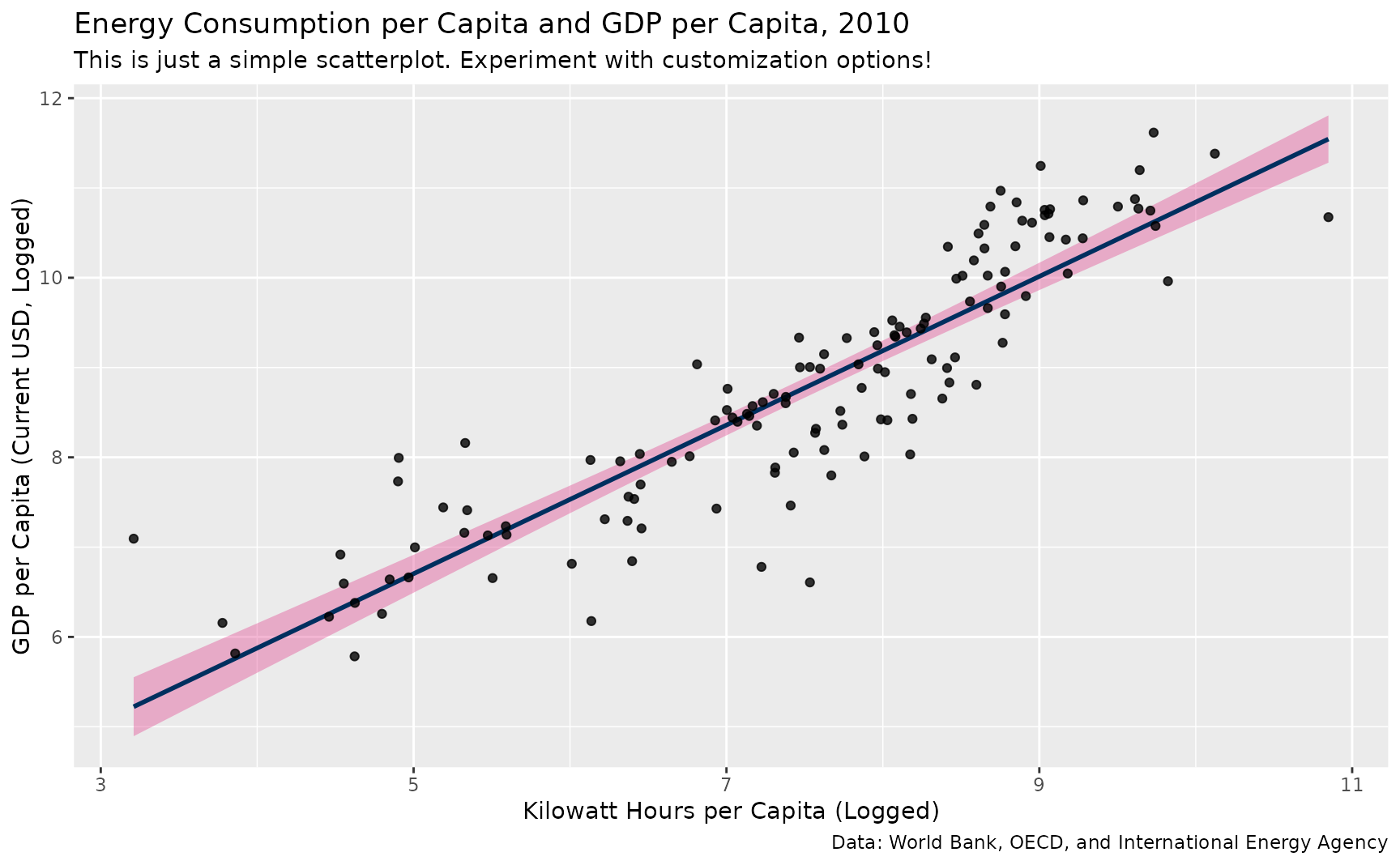example_plot() allows you to experiment with some ggplot2 themes, like those in this package, by seeing them applied to some example plots.

## Usage

example_plot(type = "bar")

type

a type

## Value

example_plot() returns a plot made in ggplot2, allowing the user to experiment with different themes to see which ones they like the best.

## Details

I'll add more here in a little bit.

Steven V. Miller

## Examples


library(ggplot2)

example_plot()example_plot(type = "faceted_line")example_plot(type = "scatter")
#> geom_smooth() using formula = 'y ~ x'